Name:    3rd Grade Math Proficient Test 6

Multiple Choice
Identify the choice that best completes the statement or answers the question.

1.

Measurement – Length, Weight, Volume – RIT 191 – 200
How long is the pencil?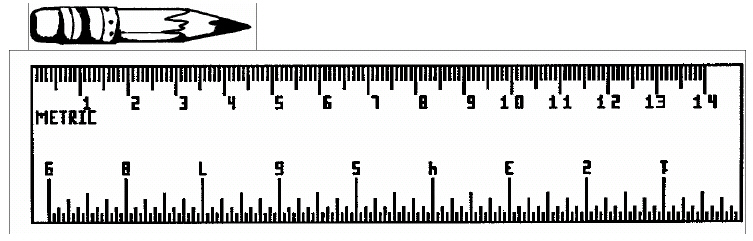a. 45 cm. d. 13 cm. b. 40 mm. e. 45 mm. c. 450 mm.

2.

Which of these units would be best to measure the length of our classroom?
 a. meters d. kilometers b. centimeters e. millimeters c. liters

3.

17 inches is equal to:
 a. 2 feet d. 17 feet b. 1 foot and 7 inches e. 12 feet and 5 inches c. 1 foot and 5 inches

4.

4 quarts is equal to: (Hint: 2 pints = 1 quart)
 a. 4 pints d. 2 pints b. 8 pints e. 1 pint c. 16 pints

5.

Measurement – Money – RIT 191 – 200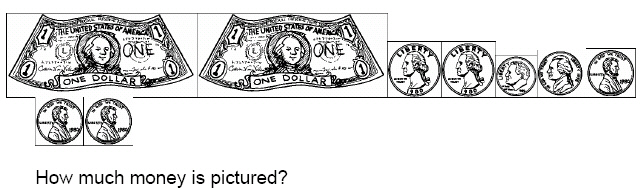a. \$3.68 d. \$2.68 b. \$8.75 e. \$2.00 c. \$15.68

6.

If Juan has \$5.40 and spends \$3.10, how much money does he have left?
 a. \$3.10 d. \$230 b. \$2.30 e. \$8.50 c. \$5.40

7.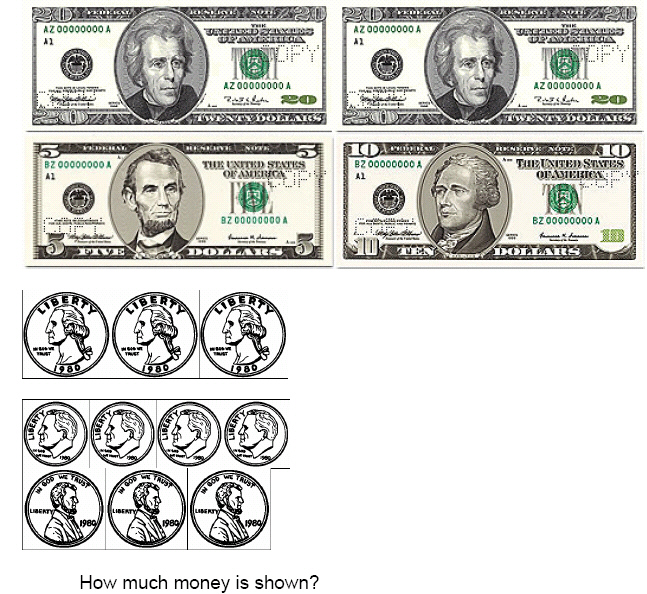a. \$56.18 d. \$55.18 b. \$81.18 e. \$5.18 c. \$55.00

8.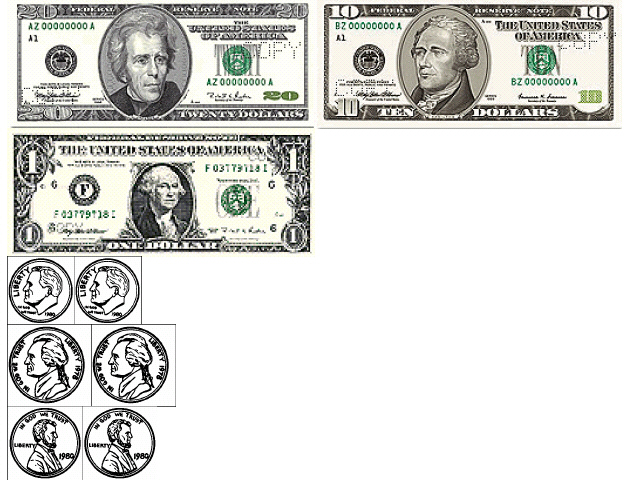How much would be left if \$9.13 was spent from the amount pictured?
 a. \$31.32 d. \$22.19 b. \$29.13 e. \$22.00 c. \$20.87

9.

Measurement – Time, Temperature – RIT 191 – 200

How many minutes are in 2 hours?
 a. 60 d. 100 b. 120 e. 200 c. 20

10.

Measurement – Angle Identification & Measure – RIT 201 – 210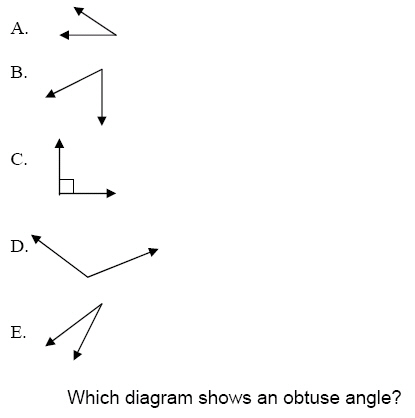a. A d. D b. B e. E c. C

11.

Measurement – Area, Perimeter, Circumference – RIT 201 – 210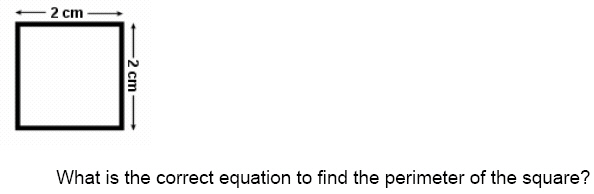a. 2cm + 2cm + 2cm = 6cm d. 2cm + 2cm = 4cm b. 2cm x 2cm = 4cm e. 2cm  2cm = 1cm c. 2cm + 2cm + 2cm + 2cm = 8cm

12.

Grass seed needed to plant 1 square meter: 1 gram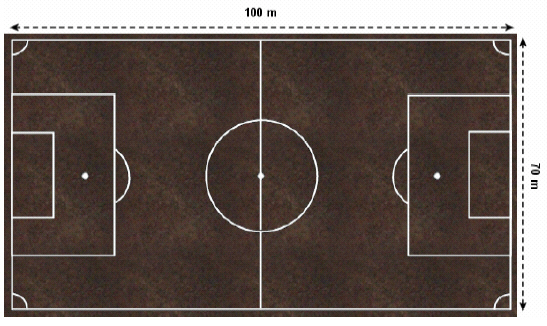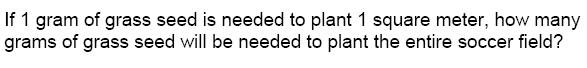a. 100 d. 5000 b. 70 e. 7000 c. 170

13.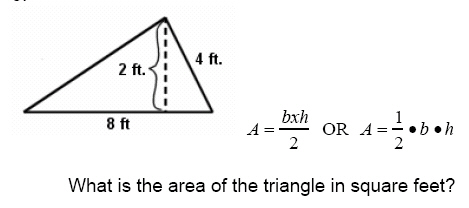a. 8 d. 10 b. 20 e. 32 c. 16

14.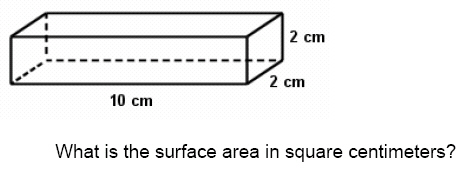a. 20 d. 14 b. 88 e. 112 c. 40

15.

Measurement – Length, Weight, Volume – RIT 201 – 210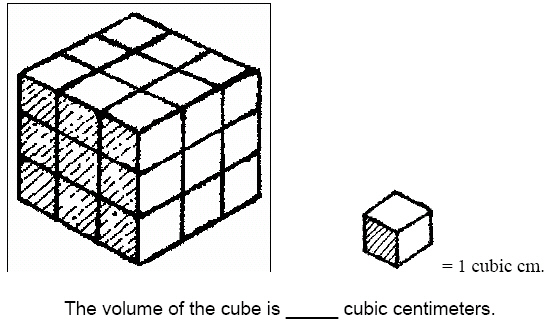a. 9 d. 27 b. 6 e. 1 c. 24

16.

947 g + 1,053 g = (Hint: 1000 g = 1 kg)
 a. 1,990 g d. 10 kg b. 894 g e. 2 kg c. 1000 g

17.

Which unit of measure would be best for measuring the area of a soccer field?
 a. meters d. inches b. centimeters e. grams c. quarts

18.

V = l w h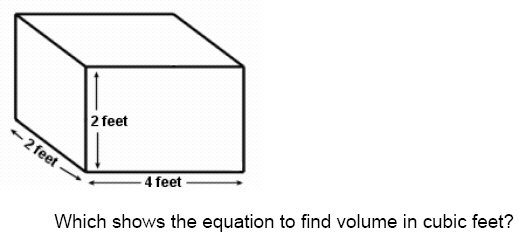a. 4 x 4 x 4 = 64 d. 2 + 2 + 2 = 6 b. 4 x 2 x 2 = 16 e. 2 x 4 + 2 = 10 c. 4 + 2 + 2 = 8

19.

Measurement – Money – RIT 201 – 210

If Yvonne had \$5.00 and spent \$3.79, what combination of bills and coins
would equal the left-over change?
 a. 1 one-dollar bill, 1 dime, and 1 penny d. 3 one-dollar bills, 3 quarters, and 4 pennies b. 3 quarters, 4 dimes, 2 nickels, and 3 pennies e. 1 one-dollar bill, 2 dimes, and 1 penny c. 2 one-dollar bills, 7 dimes, and 9 pennies

20.

If Nicholas has \$3.87 already and earns \$7.92, how much does he have
altogether?
 a. \$1,179.00 d. \$10.79 b. \$4.05 e. \$11.95 c. \$11.79